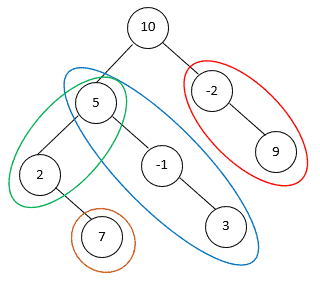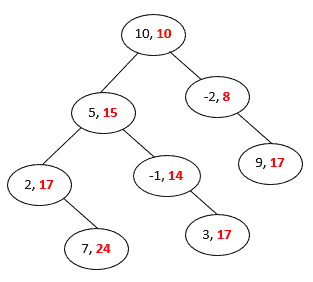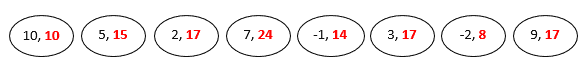## 1. Introduction

A binary tree is a hierarchical data structure in which each node has at most two children. Each node of a binary tree has 3 elements: a data element to hold an integer value and two children pointers to point its left and right children. In this tutorial, we’ll solve a binary tree path sum problem with the pre-order tree traversal algorithm.

## 2. Binary Tree Path Sum Problem

Given thenode of a binary tree and an integer, we want to print all paths where the sum of the values along each path equals. The path does not need to start at thenode or end with a leaf node. However, it must go downwards. That is, we traverse the path from parent nodes to child nodes.

Also, the path can be a single node whose data value is. For example, we have 4 paths that satisfy theofin the following binary tree:In the extreme case, we can have a binary tree whose node values are alland ouris also. In this case, we need to print out all possible paths that go downwards.

## 3. Pre-Order Path Sum Sequence

For each tree node, we can record the path sum value from thenode to this node:we can use the pre-order tree traversal to calculate the path sum for each tree node. In a pre-order traversal, we first visit thenode. Then, we visit the left and right subtrees. Therefore, we can guarantee a downward path between any two nodes in a pre-order traversal sequence. For example, the pre-order path sum sequence of the example binary tree is:Each element of the pre-order traversal sequence contains two values: the current tree node and the path sum from thenode to the current tree node:We can use a recursive pre-order tree traversal algorithm to construct the path sum sequence:In this algorithm, we start with thenode. We first put its path sum node into the sequence. Then, we recursively visit its left and right children. In each recursive call, we add the current tree node’s data into the accumulated path sum value. Therefore, thefield contains the path sum value from thenode to the current tree node.

If the binary tree containsnodes, the overall running time of this algorithm issince we visit each node only once.

## 4. Print All Paths with Target Sum

We can calculate the path sum between any two tree nodes in constant time with the pre-order path sum sequence. For any two nodes in the path sum sequence with indexesand(), the path sum between the two nodes is. For example, we can calculate the path sum between node 5 and node 3 in the example tree as.

Based on this formula, we can find all paths whose path sum values are equal to:In this algorithm, we search all pairs of tree nodes as the path start and end nodes. For each pair of nodes, we calculate its path sum and compare the result with. If they are the same, we then print the path between these two nodes.

We can use a top-down approach to print the path between any two tree nodes:This algorithm also uses the pre-order traversal to search the binary tree. In each recursive call, we first append the current node into the path. We stop the searching when we locate thenode. Otherwise, we continue the search on the current node’s left and right subtree.

To find all paths, we use a nested loop to search all possible pairs of binary tree nodes. This takestime. Also, when we find a path whose path sum is, we need an extratime to print the path. Therefore, the overall time complexity of the algorithm is.

## 5. Conclusion

In this tutorial, we showed how to construct a binary tree path sum sequence in linear time. Based on this sequence, we can print all paths withintime.